博文目录
1. 概述2. 梯度下降算法2.1 场景假设2.2 梯度下降2.2.1 微分2.2.2 梯度
2.3 数学解释2.3.1 α2.3.2 梯度要乘以一个负号

3. 实例3.1 单变量函数的梯度下降3.2 多变量函数的梯度下降
4. 代码实现4. 1 场景分析4. 2 代码
5. 小结

1. 概述
2. 梯度下降算法
2.1 场景假设
梯度下降法的基本思想可以类比为一个下山的过程。 假设这样一个场景：一个人被困在山上，需要从山上下来(找到山的最低点，也就是山谷)。但此时山上的浓雾很大，导致可视度很低；因此，下山的路径就无法确定，必须利用自己周围的信息一步一步地找到下山的路。这个时候，便可利用梯度下降算法来帮助自己下山。怎么做呢，首先以他当前的所处的位置为基准，寻找这个位置最陡峭的地方，然后朝着下降方向走一步，然后又继续以当前位置为基准，再找最陡峭的地方，再走直到最后到达最低处；同理上山也是如此，只是这时候就变成梯度上升算法了2.2 梯度下降
梯度下降的基本过程就和下山的场景很类似。
首先，我们有一个可微分的函数。这个函数就代表着一座山。我们的目标就是找到这个函数的最小值，也就是山底。根据之前的场景假设，最快的下山的方式就是找到当前位置最陡峭的方向，然后沿着此方向向下走，对应到函数中，就是找到给定点的梯度 ，然后朝着梯度相反的方向，就能让函数值下降的最快！因为梯度的方向就是函数之变化最快的方向(在后面会详细解释) 所以，我们重复利用这个方法，反复求取梯度，最后就能到达局部的最小值，这就类似于我们下山的过程。而求取梯度就确定了最陡峭的方向，也就是场景中测量方向的手段。那么为什么梯度的方向就是最陡峭的方向呢？接下来，我们从微分开始讲起：
2.2.1 微分
看待微分的意义，可以有不同的角度，最常用的两种是：
函数图像中，某点的切线的斜率函数的变化率 几个微分的例子：
1.单变量的微分，函数只有一个变量时

d

(

x

2

)

d

x

=

2

x

\frac{d(x^2)}{dx}=2x

d

(

−

2

y

5

)

d

y

=

−

10

y

4

\frac{d(-2y^5)}{dy}=-10y^4

d

(

5

−

θ

)

2

d

θ

=

−

2

(

5

−

θ

)

\frac{d(5-\theta )^2}{d\theta}=-2(5-\theta)

2.多变量的微分，当函数有多个变量的时候，即分别对每个变量进行求微分

∂

∂

x

(

x

2

y

2

)

=

2

x

y

2

\frac{\partial}{\partial x}(x^2y^2) = 2xy^2

∂

∂

y

(

−

2

y

5

+

z

2

)

=

−

10

y

4

\frac{\partial}{\partial y}(-2y^5+z^2) = -10y^4

∂

∂

θ

2

(

5

θ

1

+

2

θ

2

−

12

θ

3

)

=

2

\frac{\partial}{\partial \theta_{2}}(5\theta_{1} + 2\theta_{2} - 12\theta_{3}) = 2

∂

∂

θ

2

(

0.55

−

(

5

θ

1

+

2

θ

2

−

12

θ

3

)

)

=

−

2

\frac{\partial}{\partial \theta_{2}}(0.55 - (5\theta_{1} + 2\theta_{2} - 12\theta_{3})) = -2

2.2.2 梯度
梯度实际上就是多变量微分的一般化。 下面这个例子：

J

(

Θ

)

=

0.55

−

(

5

θ

1

+

2

θ

2

−

12

θ

3

)

J(\Theta ) = 0.55 - (5\theta_{1} + 2\theta_{2} - 12\theta_{3})

▽

J

(

Θ

)

=

<

∂

J

∂

θ

1

,

∂

J

∂

θ

2

,

∂

J

∂

θ

3

>

=

(

−

5

,

−

2

,

12

)

\triangledown J(\Theta ) = \left < \frac{\partial J}{\partial \theta_{1}}, \frac{\partial J}{\partial \theta_{2}},\frac{\partial J}{\partial \theta_{3}} \right > =(-5,-2,12)

我们可以看到，梯度就是分别对每个变量进行微分，然后用逗号分割开，梯度是用<>包括起来，说明梯度其实一个向量。
梯度是微积分中一个很重要的概念，之前提到过梯度的意义
在单变量的函数中，梯度其实就是函数的微分，代表着函数在某个给定点的切线的斜率在多变量函数中，梯度是一个向量，向量有方向，梯度的方向就指出了函数在给定点的上升最快的方向
这也就说明了为什么我们需要千方百计的求取梯度！我们需要到达山底，就需要在每一步观测到此时最陡峭的地方，梯度就恰巧告诉了我们这个方向。梯度的方向是函数在给定点上升最快的方向，那么梯度的反方向就是函数在给定点下降最快的方向，这正是我们所需要的。所以我们只要沿着梯度的方向一直走，就能走到局部的最低点！
2.3 数学解释
首先给出数学公式：

Θ

1

=

Θ

0

+

α

▽

J

(

Θ

)

→

e

v

a

l

u

a

t

e

d

a

t

Θ

0

{\color{Red} \Theta^1} = {\color{Blue} \Theta^0} + {\color{Green} \alpha} {\color{Purple} \triangledown J(\Theta)}\rightarrow evaluated at \Theta^0

此公式的意义是：J是关于Θ的一个函数，我们当前所处的位置为Θ0点，要从这个点走到J的最小值点，也就是山底。首先我们先确定前进的方向，也就是梯度的反向，然后走一段距离的步长，也就是α，走完这个段步长，就到达了Θ1这个点！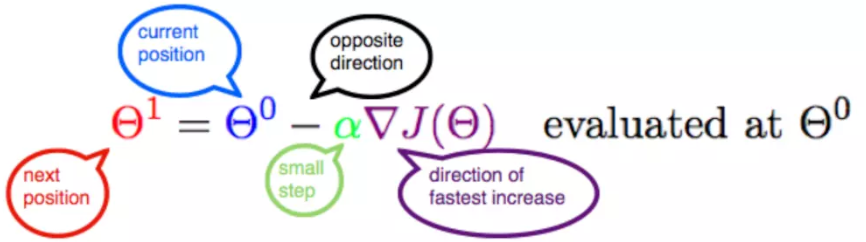2.3.1 α
α在梯度下降算法中被称作为学习率或者步长，意味着我们可以通过α来控制每一步走的距离，以保证不要步子跨的太大扯着蛋，哈哈，其实就是不要走太快，错过了最低点。同时也要保证不要走的太慢，导致太阳下山了，还没有走到山下。所以α的选择在梯度下降法中往往是很重要的！α不能太大也不能太小，太小的话，可能导致迟迟走不到最低点，太大的话，会导致错过最低点！
2.3.2 梯度要乘以一个负号
梯度前加一个负号，就意味着朝着梯度相反的方向前进！我们在前文提到，梯度的方向实际就是函数在此点上升最快的方向！而我们需要朝着下降最快的方向走，自然就是负的梯度的方向，所以此处需要加上负号；那么如果时上坡，也就是梯度上升算法，当然就不需要添加负号了。
3. 实例
我们已经基本了解了梯度下降算法的计算过程，那么我们就来看几个梯度下降算法的小实例，首先从单变量的函数开始，然后介绍多变量的函数。
3.1 单变量函数的梯度下降
我们假设有一个单变量的函数

J

(

θ

)

=

θ

2

J(\theta) = \theta^2

函数的微分，直接求导就可以得到

J

′

(

θ

)

=

2

θ

J'(\theta) = 2\theta

初始化，也就是起点，起点可以随意的设置，这里设置为1

θ

0

=

1

\theta^0 = 1

学习率也可以随意的设置，这里设置为0.4

α

=

0.4

\alpha = 0.4

根据梯度下降的计算公式

Θ

1

=

Θ

0

+

α

▽

J

(

Θ

)

→

e

v

a

l

u

a

t

e

d

a

t

Θ

0

{\color{Red} \Theta^1} = {\color{Blue} \Theta^0} + {\color{Green} \alpha} {\color{Purple} \triangledown J(\Theta)}\rightarrow evaluated at \Theta^0

我们开始进行梯度下降的迭代计算过程：

θ

0

=

1

\theta^0 = 1

θ

1

=

θ

0

−

α

∗

J

′

(

θ

0

)

=

1

−

0.4

∗

2

=

0.2

\theta^1 = \theta^0 - \alpha*J'(\theta^0)=1 - 0.4*2 = 0.2

θ

2

=

θ

1

−

α

∗

J

′

(

θ

1

)

=

0.2

−

0.4

∗

0.4

=

0.04

\theta^2 = \theta^1 - \alpha*J'(\theta^1)= 0.2 - 0.4*0.4=0.04

θ

3

=

0.008

\theta^3 = 0.008

θ

4

=

0.0016

\theta^4 = 0.0016

如图，经过四次的运算，也就是走了四步，基本就抵达了函数的最低点，也就是山底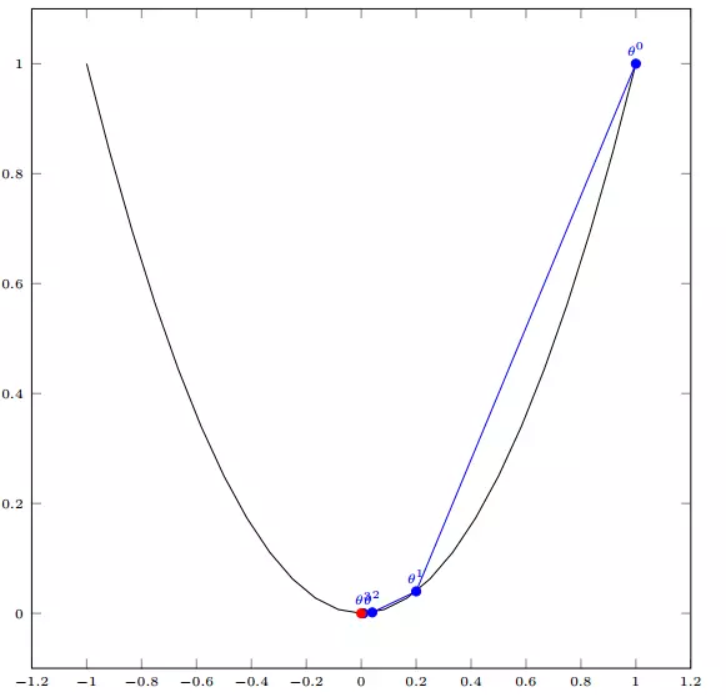3.2 多变量函数的梯度下降
我们假设有一个目标函数

J

(

Θ

)

=

θ

1

2

+

θ

2

2

J(\Theta) = \theta_{1}^2 + \theta_{2}^2

现在要通过梯度下降法计算这个函数的最小值。我们通过观察就能发现最小值其实就是 (0，0)点。但是接下来，我们会从梯度下降算法开始一步步计算到这个最小值！ 我们假设初始的起点为：

Θ

0

=

(

1

,

3

)

\Theta^0 = (1, 3)

初始的学习率为：

α

=

0.1

\alpha = 0.1

函数的梯度为：

▽

J

(

Θ

)

=

<

2

θ

1

,

2

θ

2

>

\triangledown J(\Theta ) = \left < 2\theta_{1},2\theta_{2} \right >

进行多次迭代：

Θ

0

=

(

1

,

3

)

\Theta^0 = (1, 3)

Θ

1

=

Θ

0

−

α

▽

J

(

Θ

)

=

(

1

,

3

)

−

0.1

∗

(

2

,

6

)

=

(

0.8

,

2.4

)

\Theta^1 = \Theta^0 - \alpha\triangledown J(\Theta ) = (1,3) - 0.1*(2, 6)=(0.8, 2.4)

Θ

2

=

(

0.8

,

2.4

)

−

0.1

∗

(

1.6

,

4.8

)

=

(

0.64

,

1.92

)

\Theta^2 = (0.8, 2.4) - 0.1*(1.6, 4.8)=(0.64, 1.92)

Θ

3

=

(

0.5124

,

1.536

)

\Theta^3 =(0.5124, 1.536)

Θ

4

=

(

0.4096

,

1.228800000000001

)

\Theta^4 =(0.4096, 1.228800000000001)

⋮

\vdots

Θ

10

=

(

0.1073741824000003

,

0.32212254720000005

)

\Theta^{10} =(0.1073741824000003, 0.32212254720000005)

⋮

\vdots

Θ

50

=

(

1.141798154164342

e

−

05

,

3.42539442494306

e

−

05

)

\Theta^{50} =(1.141798154164342e^{-05}, 3.42539442494306e^{-05})

⋮

\vdots

Θ

100

=

(

1.6296287810675902

e

−

10

,

4.8888886343202771

e

−

10

)

\Theta^{100} =(1.6296287810675902e^{-10}, 4.8888886343202771e^{-10})

我们发现，已经基本靠近函数的最小值点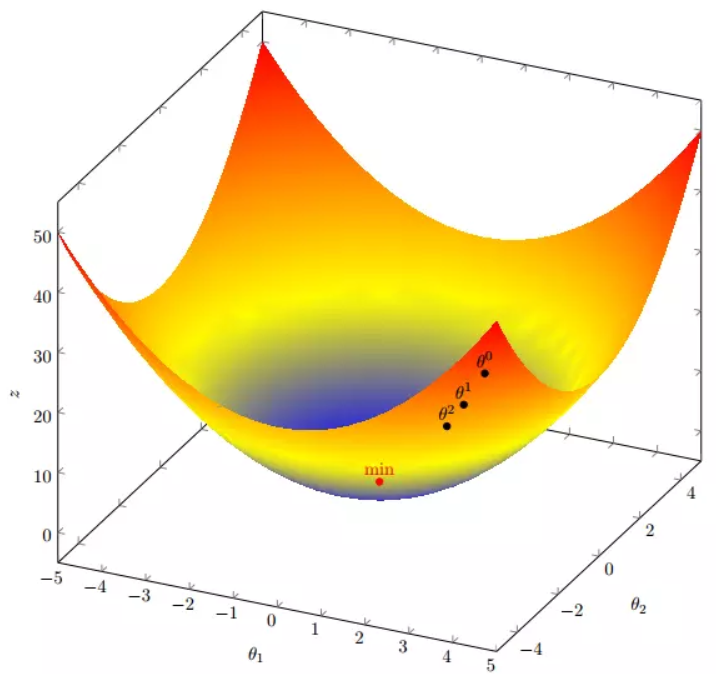4. 代码实现
4. 1 场景分析
下面我们将用python实现一个简单的梯度下降算法。场景是一个简单的线性回归的例子：假设现在我们有一系列的点，如下图所示：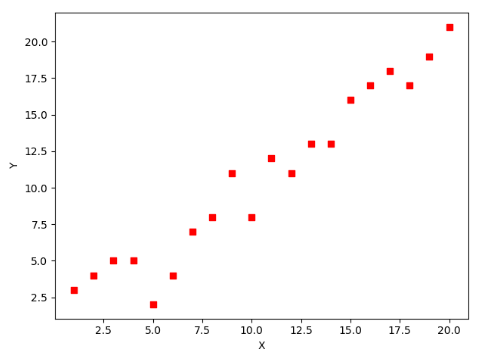我们将用梯度下降法来拟合出这条直线！
首先，我们需要定义一个代价函数，在此我们选用均方误差代价函数（也称平方误差代价函数）

J

(

Θ

)

=

1

2

m

∑

i

=

1

m

(

h

θ

(

x

(

i

)

)

−

y

(

i

)

)

2

J(\Theta) = \frac{1}{2m}\sum_{i=1}^{m}(h_{\theta}(x^{(i)})-y^{(i)})^2

此公式中
m是数据集中数据点的个数，也就是样本数½是一个常量，这样是为了在求梯度的时候，二次方乘下来的2就和这里的½抵消了，自然就没有多余的常数系数，方便后续的计算，同时对结果不会有影响y 是数据集中每个点的真实y坐标的值，也就是类标签h 是我们的预测函数（假设函数），根据每一个输入x，根据Θ 计算得到预测的y值，即

h

Θ

(

x

(

i

)

)

=

Θ

0

+

Θ

1

x

1

(

i

)

h_{\Theta}(x^{(i)}) = \Theta_{0} + \Theta_{1}x_{1}^{(i)}

我们可以根据代价函数看到，代价函数中的变量有两个，所以是一个多变量的梯度下降问题，求解出代价函数的梯度，也就是分别对两个变量进行微分

▽

J

(

Θ

)

=

<

δ

J

δ

Θ

0

,

δ

J

δ

Θ

1

>

\triangledown J(\Theta ) = \left < \frac{\delta J}{\delta \Theta_{0}}, \frac{\delta J}{\delta \Theta_{1}} \right >

δ

J

δ

Θ

0

=

1

m

∑

i

=

1

m

(

h

Θ

(

x

(

i

)

)

−

y

(

i

)

)

\frac{\delta J}{\delta \Theta_{0}} = \frac{1}{m}\sum_{i=1}^{m}(h_{\Theta}(x^{(i)})-y^{(i)})

δ

J

δ

Θ

1

=

1

m

∑

i

=

1

m

(

h

Θ

(

x

(

i

)

)

−

y

(

i

)

)

x

1

(

i

)

\frac{\delta J}{\delta \Theta_{1}} = \frac{1}{m}\sum_{i=1}^{m}(h_{\Theta}(x^{(i)})-y^{(i)})x_{1}^{(i)}

明确了代价函数和梯度，以及预测的函数形式。我们就可以开始编写代码了。但在这之前，需要说明一点，就是为了方便代码的编写，我们会将所有的公式都转换为矩阵的形式，python中计算矩阵是非常方便的，同时代码也会变得非常的简洁。 为了转换为矩阵的计算，我们观察到预测函数的形式

h

Θ

(

x

(

i

)

)

=

Θ

0

+

Θ

1

x

(

i

)

h_{\Theta}(x^{(i)}) = \Theta_{0} + \Theta_{1}x^{(i)}

我们有两个变量，为了对这个公式进行矩阵化，我们可以给每一个点x增加一维，这一维的值固定为1，这一维将会乘到Θ0上。这样就方便我们统一矩阵化的计算

(

x

1

(

i

)

,

y

(

i

)

)

→

(

x

0

(

i

)

,

x

1

(

i

)

,

y

(

i

)

)

w

i

t

h

x

0

(

i

)

=

1

∀

i

(x_{1}^{(i)},y^{(i)})\rightarrow (x_{0}^{(i)},x_{1}^{(i)},y^{(i)}) with x_{0}^{(i)} = 1 \forall _{i}

然后我们将代价函数和梯度转化为矩阵向量相乘的形式

J

(

Θ

)

=

1

2

m

(

X

Θ

−

y

⃗

)

T

(

X

Θ

−

y

⃗

)

J(\Theta) = \frac{1}{2m}(X\Theta - \vec{y})^{T}(X\Theta - \vec{y})

▽

J

(

Θ

)

=

1

m

X

T

(

X

Θ

−

y

⃗

)

)

\triangledown J(\Theta) = \frac{1}{m}X^{T}(X\Theta - \vec{y}))

4. 2 代码
首先，我们需要定义数据集和学习率
#!/usr/bin/env python3
# -*- coding: utf-8 -*-
# @Time    : 2019/1/21 21:06
# @Author  : Arrow and Bullet
# @Software: PyCharm
# @Blog    ：https://blog.csdn.net/qq_41800366

from numpy import *

# 数据集大小 即20个数据点
m = 20
# x的坐标以及对应的矩阵
X0 = ones((m, 1))  # 生成一个m行1列的向量，也就是x0，全是1
X1 = arange(1, m+1).reshape(m, 1)  # 生成一个m行1列的向量，也就是x1，从1到m
X = hstack((X0, X1))  # 按照列堆叠形成数组，其实就是样本数据
# 对应的y坐标
y = np.array([
3, 4, 5, 5, 2, 4, 7, 8, 11, 8, 12,
11, 13, 13, 16, 17, 18, 17, 19, 21
]).reshape(m, 1)
# 学习率
alpha = 0.01

接下来我们以矩阵向量的形式定义代价函数和代价函数的梯度
# 定义代价函数
def cost_function(theta, X, Y):
diff = dot(X, theta) - Y  # dot() 数组需要像矩阵那样相乘，就需要用到dot()
return (1/(2*m)) * dot(diff.transpose(), diff)

# 定义代价函数对应的梯度函数
diff = dot(X, theta) - Y
return (1/m) * dot(X.transpose(), diff)

最后就是算法的核心部分，梯度下降迭代计算
# 梯度下降迭代
theta = array([1, 1]).reshape(2, 1)
theta = theta - alpha * gradient
return theta

print('optimal:', optimal)
print('cost function:', cost_function(optimal, X, Y))

当梯度小于1e-5时，说明已经进入了比较平滑的状态，类似于山谷的状态，这时候再继续迭代效果也不大了，所以这个时候可以退出循环！ 运行代码，计算得到的结果如下：
print('optimal:', optimal)  # 结果 [[0.51583286][0.96992163]]
print('cost function:', cost_function(optimal, X, Y))  # 1.014962406233101

通过matplotlib画出图像，
# 根据数据画出对应的图像
def plot(X, Y, theta):
import matplotlib.pyplot as plt
ax = plt.subplot(111)  # 这是我改的
ax.scatter(X, Y, s=30, c="red", marker="s")
plt.xlabel("X")
plt.ylabel("Y")
x = arange(0, 21, 0.2)  # x的范围
y = theta + theta*x
ax.plot(x, y)
plt.show()

plot(X1, Y, optimal)

所拟合出的直线如下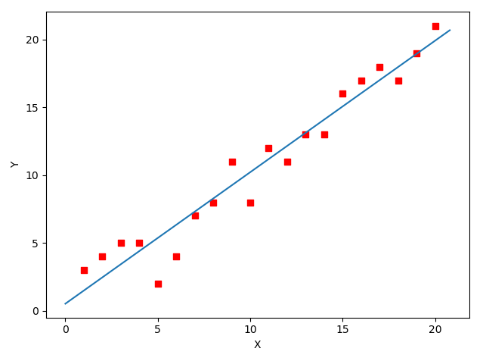全部代码如下，大家有兴趣的可以复制下来跑一下看一下结果：
#!/usr/bin/env python3
# -*- coding: utf-8 -*-
# @Time    : 2019/1/21 21:06
# @Author  : Arrow and Bullet
# @Software: PyCharm
# @Blog    ：https://blog.csdn.net/qq_41800366

from numpy import *

# 数据集大小 即20个数据点
m = 20
# x的坐标以及对应的矩阵
X0 = ones((m, 1))  # 生成一个m行1列的向量，也就是x0，全是1
X1 = arange(1, m+1).reshape(m, 1)  # 生成一个m行1列的向量，也就是x1，从1到m
X = hstack((X0, X1))  # 按照列堆叠形成数组，其实就是样本数据
# 对应的y坐标
Y = array([
3, 4, 5, 5, 2, 4, 7, 8, 11, 8, 12,
11, 13, 13, 16, 17, 18, 17, 19, 21
]).reshape(m, 1)
# 学习率
alpha = 0.01

# 定义代价函数
def cost_function(theta, X, Y):
diff = dot(X, theta) - Y  # dot() 数组需要像矩阵那样相乘，就需要用到dot()
return (1/(2*m)) * dot(diff.transpose(), diff)

# 定义代价函数对应的梯度函数
diff = dot(X, theta) - Y
return (1/m) * dot(X.transpose(), diff)

# 梯度下降迭代
theta = array([1, 1]).reshape(2, 1)
theta = theta - alpha * gradient
return theta

print('optimal:', optimal)
print('cost function:', cost_function(optimal, X, Y))

# 根据数据画出对应的图像
def plot(X, Y, theta):
import matplotlib.pyplot as plt
ax = plt.subplot(111)  # 这是我改的
ax.scatter(X, Y, s=30, c="red", marker="s")
plt.xlabel("X")
plt.ylabel("Y")
x = arange(0, 21, 0.2)  # x的范围
y = theta + theta*x
ax.plot(x, y)
plt.show()

plot(X1, Y, optimal)

5. 小结
至此，就基本介绍完了梯度下降法的基本思想和算法流程，并且用python实现了一个简单的梯度下降算法拟合直线的案例！ 最后，我们回到文章开头所提出的场景假设: 这个下山的人实际上就代表了反向传播算法，下山的路径其实就代表着算法中一直在寻找的参数Θ，山上当前点的最陡峭的方向实际上就是代价函数在这一点的梯度方向，场景中观测最陡峭方向所用的工具就是微分 。在下一次观测之前的时间就是有我们算法中的学习率α所定义的。 可以看到场景假设和梯度下降算法很好的完成了对应！
本文部分内容来自一位前辈，非常感谢分享！谢谢！
展开全文梯度下降
• 本文内容为东北大学数值分析国家精品慕课课程的课程讲义,将其整理为OneNote笔记同时添加了本人上课时的课堂笔记,且主页中的思维导图就是根据课件内容整理而来, 为了方便大家和自己查看，特将此上传到CSDN博文中, ...
本文内容为东北大学数值分析国家精品慕课课程的课程讲义, 将其整理为OneNote笔记同时添加了本人上课时的课堂笔记, 且主页中的思维导图就是根据课件内容整理而来,
为了方便大家和自己查看，特将此上传到CSDN博文中, 源文件已经上传到我的资源中,有需要的可以去看看,
我主页中的思维导图中内容大多从我的笔记中整理而来,相应技巧可在笔记中查找原题, 有兴趣的可以去 我的主页 了解更多计算机学科的精品思维导图整理
本文可以转载，但请注明来处，觉得整理的不错的小伙伴可以点赞关注支持一下哦！
博客中思维导图的高清PDF版本,可关注公众号 一起学计算机 点击 资源获取 获得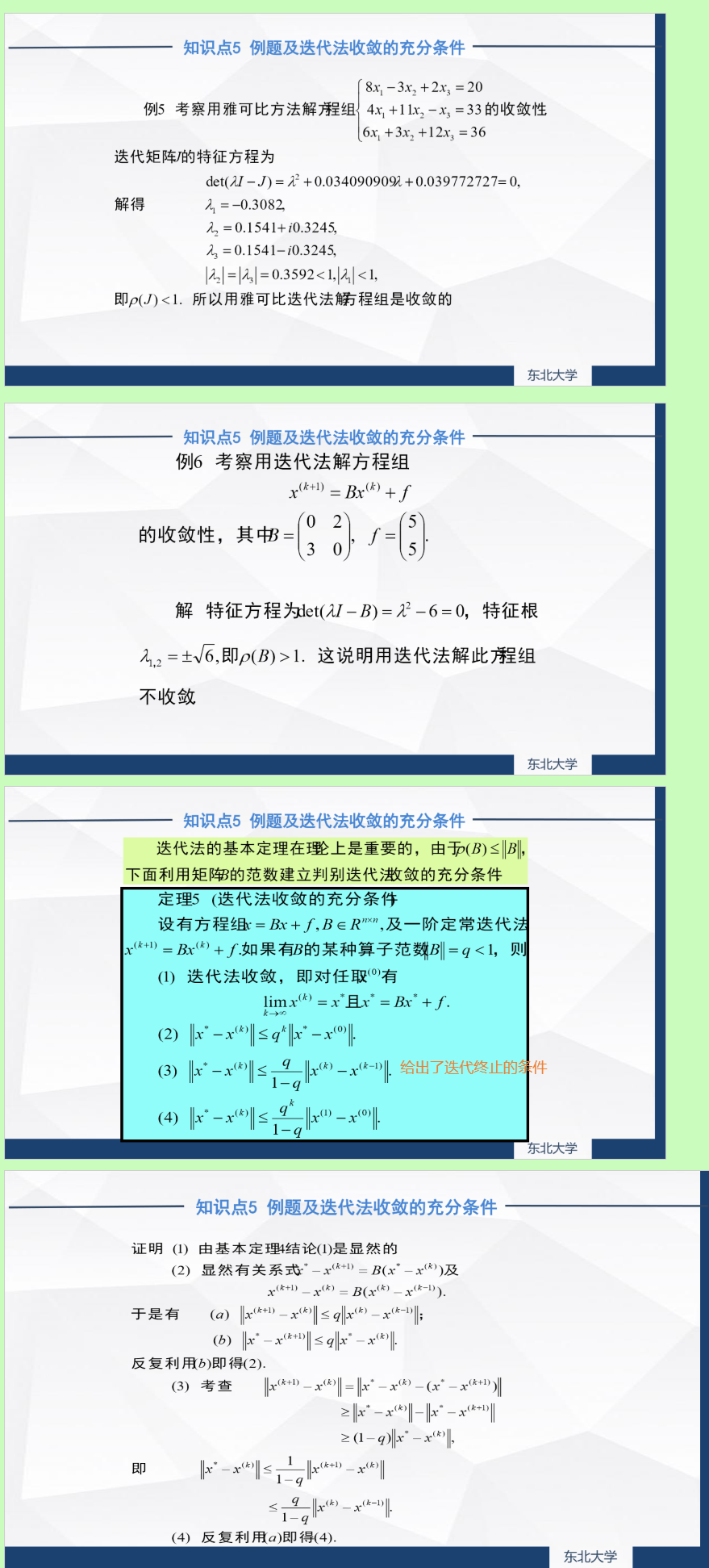感觉作者写的不错的, 别忘了点赞关注加收藏哦(一键三连)!你的支持会带给我极大的动力, 写出更多优秀文章!文章到这里就结束了, 感谢你的认真观看, 为了感谢读者们, 我把我一直以来整理的各种计算机相关/考研相关精品思维导图/力扣算法讲解/面试资料/各种实用软件工具分享给大家(并且会持续更新哦!), 希望能够帮助到你们.
关注公众号 一起学计算机 点击 资源获取 即可获得所有资源, 包含的资源如下图, 其中具体资源的相关讲解和各种软件的使用可以查看下面相应的文章.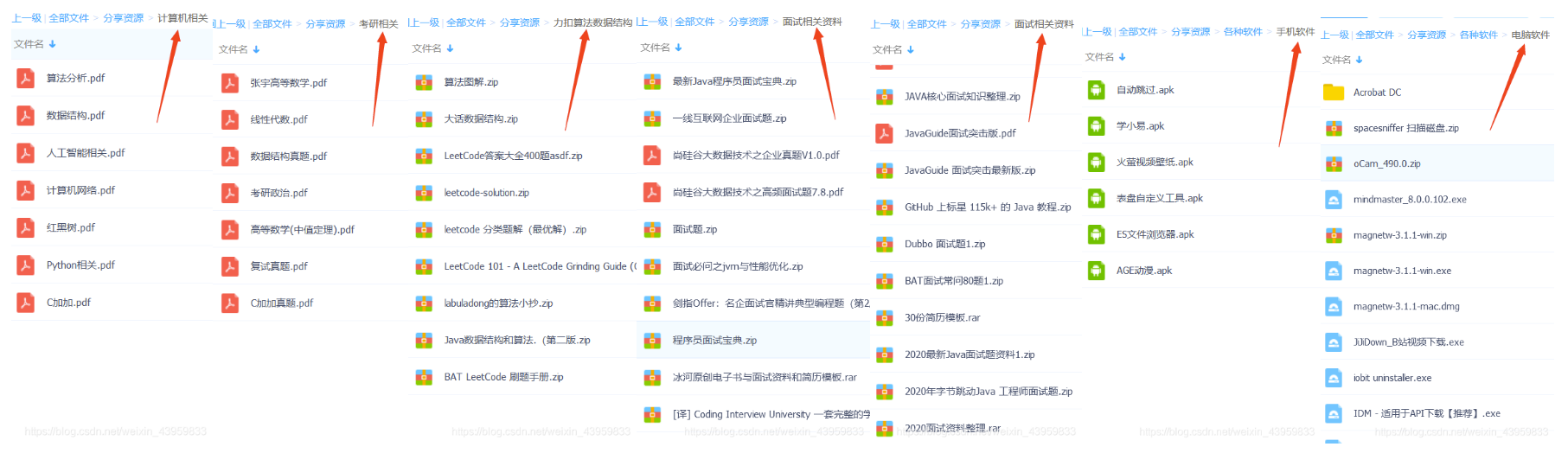我的更多精彩文章链接, 欢迎查看
各种电脑/软件/生活/音乐/动漫/电影技巧汇总(你肯定能够找到你需要的使用技巧)
力扣算法刷题 根据思维导图整理笔记快速记忆算法重点内容

经典动漫全集目录 精彩剧集
海贼王 动漫 全集目录 分章节 精彩打斗剧集 思维导图整理
火影忍者 动漫 全集目录 分章节 精彩打斗剧集 思维导图整理
死神 动漫 全集目录 分章节 精彩打斗剧集 思维导图整理

计算机专业知识 思维导图整理
最值得收藏的 Python 全部知识点思维导图整理, 附带常用代码/方法/库/数据结构/常见错误/经典思想(持续更新中)
最值得收藏的 C++ 全部知识点思维导图整理(清华大学郑莉版), 东南大学软件工程初试906科目
最值得收藏的 计算机网络 全部知识点思维导图整理(王道考研), 附带经典5层结构的中英对照和框架简介
最值得收藏的 算法分析与设计 全部知识点思维导图整理(北大慕课课程)
最值得收藏的 数据结构 全部知识点思维导图整理(王道考研), 附带经典题型整理
最值得收藏的 人工智能导论 全部知识点思维导图整理(王万良慕课课程)
最值得收藏的 数值分析 全部知识点思维导图整理(东北大学慕课课程)
最值得收藏的 数字图像处理 全部知识点思维导图整理(武汉大学慕课课程)
红黑树 一张导图解决红黑树全部插入和删除问题 包含详细操作原理 情况对比
各种常见排序算法的时间/空间复杂度 是否稳定 算法选取的情况 改进 思维导图整理

人工智能课件  算法分析课件  Python课件  数值分析课件  机器学习课件 图像处理课件

考研相关科目 知识点 思维导图整理
考研经验--东南大学软件学院软件工程(这些基础课和专业课的各种坑和复习技巧你应该知道)
东南大学 软件工程 906 数据结构 C++ 历年真题 思维导图整理
东南大学 软件工程 复试3门科目历年真题 思维导图整理
最值得收藏的 考研高等数学 全部知识点思维导图整理(张宇, 汤家凤), 附带做题技巧/易错点/知识点整理
最值得收藏的 考研线性代数 全部知识点思维导图整理(张宇, 汤家凤), 附带惯用思维/做题技巧/易错点整理
高等数学 中值定理 一张思维导图解决中值定理所有题型
考研思修 知识点 做题技巧 同类比较 重要会议 1800易错题 思维导图整理
考研近代史 知识点 做题技巧 同类比较 重要会议 1800易错题 思维导图整理
考研马原 知识点 做题技巧 同类比较 重要会议 1800易错题 思维导图整理

考研数学课程笔记  考研英语课程笔记  考研英语单词词根词缀记忆  考研政治课程笔记

Python相关技术 知识点 思维导图整理
Numpy常见用法全部OneNote笔记     全部笔记思维导图整理
Pandas常见用法全部OneNote笔记     全部笔记思维导图整理
Matplotlib常见用法全部OneNote笔记  全部笔记思维导图整理
PyTorch常见用法全部OneNote笔记    全部笔记思维导图整理
Scikit-Learn常见用法全部OneNote笔记  全部笔记思维导图整理

Java相关技术/ssm框架全部笔记
Spring  springmvc  Mybatis  jsp

科技相关 小米手机
小米 红米 历代手机型号大全 发布时间 发布价格
常见手机品牌的各种系列划分及其特点
历代CPU和GPU的性能情况和常见后缀的含义 思维导图整理
展开全文数值分析 迭代法 充分条件
• 对于给定的正整数x与允许误差e，令变量y取任意正实数值，如另y=x; 如果yy与x足够接近，即|yy-x|<e,计算结束并把y作为结果； 否则，取z=(y+x/y)/2; 将z作为y的新值，回到步骤1 # 首先，编写代码是比较容易实现...
对于给定的正整数x与允许误差e，令变量y取任意正实数值，如另y=x;
如果yy与x足够接近，即|yy-x|<e,计算结束并把y作为结果；否则，取z=(y+x/y)/2;将z作为y的新值，回到步骤1
# 首先，编写代码是比较容易实现的

def get_sqrt(x,e=10**(-6)):
y=x
while abs(y*y-x)>e:
z=(y+x/y)/2.0
y=z
return y

print(get_sqrt(4))
print(get_sqrt(5))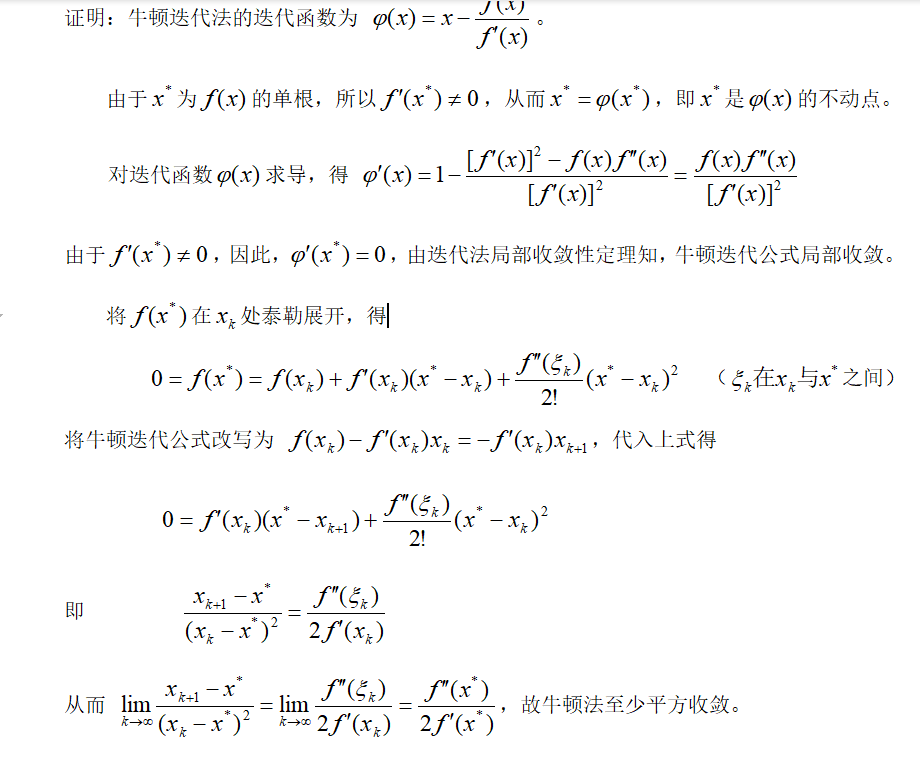问题咨询请扫码：展开全文算法
• 可能收敛局部最小值, 在大规模数据集上收敛较慢 对于异常点、离群点敏感 使用数据类型 : 数值型数据 k-means聚类算法实现 案例描述 我们假设这样的一个案例需求：某公司发布一批新型手机，根据客户...


一步步教你轻松学K-means聚类算法

阅读目录
目录聚类K-means（k均值）聚类算法案例描述从文件加载数据集计算两个向量的欧氏距离构建一个包含 K 个随机质心的集合K-Means 聚类算法分析数据：聚类可视化结果讨论与分析算法描述二分 K-Means 聚类算法伪代码二分 K-Means 聚类算法代码测试二分 KMeans 聚类算法加载数据集训练k-means算法模型可视化结果聚类结果

一步步教你轻松学K-means聚类算法

(
白宁超
2018年9月13日09:10:33)

导读：k-均值算法（英文：k-means clustering），属于比较常用的算法之一，文本首先介绍聚类的理论知识包括什么是聚类、聚类的应用、聚类思想、聚类优缺点等等；然后通过k-均值聚类案例实现及其可视化有一个直观的感受，针对算法模型进行分析和结果优化提出了二分k-means算法。最后我们调用机器学习库函数，很短的代码完成聚类算法。（本文原创，转载必须注明出处： 一步步教你轻松学K-means聚类算法

目录
1 机器学习：一步步教你轻松学KNN模型算法
2 机器学习：一步步教你轻松学决策树算法
3 机器学习：一步步教你轻松学朴素贝叶斯模型算法理论篇1
4 机器学习：一步步教你轻松学朴素贝叶斯模型实现篇2
5 机器学习：一步步教你轻松学朴素贝叶斯模型算法Sklearn深度篇3
6 机器学习：一步步教你轻松学逻辑回归模型算法
7 机器学习：一步步教你轻松学K-means聚类算法
8 机器学习：一步步教你轻松学关联规则Apriori算法
9 机器学习： 一步步教你轻松学支持向量机SVM算法之理论篇1
10 机器学习： 一步步教你轻松学支持向量机SVM算法之案例篇2
11 机器学习： 一步步教你轻松学主成分分析PCA降维算法
12 机器学习： 一步步教你轻松学支持向量机SVM降维算法
更多文章请点击这里>>
理论介绍

聚类

什么是聚类

统计数据分析的一门技术，在许多领域受到广泛应用，包括机器学习，数据挖掘，模式识别，图像分析以及生物信息。聚类是把相似的对象通过静态分类的方法分成不同的组别或者更多的子集（subset），这样让在同一个子集中的成员对象都有相似的一些属性，常见的包括在坐标系中更加短的空间距离等。

聚类的应用

在商务上，聚类能帮助市场分析人员从客户基本库中发现不同的客户群，并且用购买模式来刻画不同的客户群的特征。在生物学上，聚类能用于推导植物和动物的分类，对基因进行分类，获得对种群中固有结构的认识。聚类在地球观测数据库中相似地区的确定，汽车保险单持有者的分组，及根据房子的类型、价值和地理位置对一个城市中房屋的分组上也可以发挥作用。聚类也能用于对Web上的文档进行分类，以发现信息。诸如此类，聚类有着广泛的实际应用。

K-means（k均值）聚类算法

什么是k-means聚类算法

k-平均算法（英文：k-means clustering）源于信号处理中的一种向量量化方法，现在则更多地作为一种聚类分析方法流行于数据挖掘领域。k-平均聚类的目的是：把 n个点划分到k个聚类中，使得每个点都属于离他最近的均值（此即聚类中心）对应的聚类，以之作为聚类的标准。k-平均聚类与k-近邻之间没有任何关系（后者是另一流行的机器学习技术）。
K-Means 是发现给定数据集的 K 个簇的聚类算法, 之所以称之为 K-均值 是因为它可以发现 K 个不同的簇, 且每个簇的中心采用簇中所含值的均值计算而成.簇个数 K 是用户指定的, 每一个簇通过其质心（centroid）, 即簇中所有点的中心来描述.聚类与分类算法的最大区别在于, 分类的目标类别已知, 而聚类的目标类别是未知的.

发展历史

虽然其思想能够追溯到1957年的Hugo Steinhaus，术语“k-均值”于1967年才被James MacQueen 首次使用。标准算法则是在1957年被Stuart Lloyd作为一种脉冲码调制的技术所提出，但直到1982年才被贝尔实验室公开出版。在1965年，E.W.Forgy发表了本质上相同的方法，所以这一算法有时被称为Lloyd-Forgy方法。更高效的版本则被Hartigan and Wong提出。

算法描述

已知观测集，其中每个观测都是一个 d-维实向量，k-平均聚类要把这 n个观测划分到k个集合中(k≤n),使得组内平方和最小。换句话说，它的目标是找到使得下式满足的聚类，

其中  是  中所有点的均值。

k-means术语

簇: 所有数据的点集合，簇中的对象是相似的。质心: 簇中所有点的中心（计算所有点的均值而来）.SSE: Sum of Sqared Error（误差平方和）, 它被用来评估模型的好坏，SSE 值越小，表示越接近它们的质心. 聚类效果越 好。由于对误差取了平方，因此更加注重那些远离中心的点（一般为边界点或离群点）。详情见kmeans的评价标准。有关 簇 和 质心 术语更形象的介绍, 请参考下图:k-means应用场景

kmeans，用于数据集内种类属性不明晰，希望能够通过数据挖掘出或自动归类出有相似特点的对象的场景。其商业界的应用场景一般为挖掘出具有相似特点的潜在客户群体以便公司能够重点研究、对症下药。
例如，在2000年和2004年的美国总统大选中，候选人的得票数比较接近或者说非常接近。任一候选人得到的普选票数的最大百分比为50.7%而最小百分比为47.9% 如果1%的选民将手中的选票投向另外的候选人，那么选举结果就会截然不同。 实际上，如果妥善加以引导与吸引，少部分选民就会转换立场。尽管这类选举者占的比例较低，但当候选人的选票接近时，这些人的立场无疑会对选举结果产生非常大的影响。如何找出这类选民，以及如何在有限的预算下采取措施来吸引他们？ 答案就是聚类（Clustering)。
那么，具体如何实施呢？首先，收集用户的信息，可以同时收集用户满意或不满意的信息，这是因为任何对用户重要的内容都可能影响用户的投票结果。然后，将这些信息输入到某个聚类算法中。接着，对聚类结果中的每一个簇（最好选择最大簇 ）， 精心构造能够吸引该簇选民的消息。最后， 开展竞选活动并观察上述做法是否有效。
另一个例子就是产品部门的市场调研了。为了更好的了解自己的用户，产品部门可以采用聚类的方法得到不同特征的用户群体，然后针对不同的用户群体可以对症下药，为他们提供更加精准有效的服务。

k-means算法思想

先随机选取K个对象作为初始的聚类中心。然后计算每个对象与各个种子聚类中心之间的距离，把每个对象分配给距离它最近的聚类中心。聚类中心以及分配给它们的对象就代表一个聚类。一旦全部对象都被分配了，每个聚类的聚类中心会根据聚类中现有的对象被重新计算。这个过程将不断重复直到满足某个终止条件。终止条件可以是以下任何一个：
没有（或最小数目）对象被重新分配给不同的聚类。没有（或最小数目）聚类中心再发生变化。误差平方和局部最小。
得到相互分离的球状聚类，在这些聚类中，均值点趋向收敛于聚类中心。 一般会希望得到的聚类大小大致相当，这样把每个观测都分配到离它最近的聚类中心（即均值点）就是比较正确的分配方案。

k-means工作流程

创建 k 个点作为起始质心（通常是随机选择）
当任意一个点的簇分配结果发生改变时（不改变时算法结束）
对数据集中的每个数据点
对每个质心
计算质心与数据点之间的距离
将数据点分配到距其最近的簇
对每一个簇, 计算簇中所有点的均值并将均值作为质心


k-means开发流程

收集数据：使用任意方法
准备数据：需要数值型数据类计算距离, 也可以将标称型数据映射为二值型数据再用于距离计算
分析数据：使用任意方法
训练算法：不适用于无监督学习，即无监督学习不需要训练步骤
测试算法：应用聚类算法、观察结果.可以使用量化的误差指标如误差平方和（后面会介绍）来评价算法的结果.
使用算法：可以用于所希望的任何应用.通常情况下, 簇质心可以代表整个簇的数据来做出决策.


k-means评价标准

k-means算法因为手动选取k值和初始化随机质心的缘故，每一次的结果不会完全一样，而且由于手动选取k值，我们需要知道我们选取的k值是否合理，聚类效果好不好，那么如何来评价某一次的聚类效果呢？也许将它们画在图上直接观察是最好的办法，但现实是，我们的数据不会仅仅只有两个特征，一般来说都有十几个特征，而观察十几维的空间对我们来说是一个无法完成的任务。因此，我们需要一个公式来帮助我们判断聚类的性能，这个公式就是SSE (Sum of Squared Error, 误差平方和 ），它其实就是每一个点到其簇内质心的距离的平方值的总和，这个数值对应kmeans函数中clusterAssment矩阵的第一列之和。 SSE值越小表示数据点越接近于它们的质心，聚类效果也越好。 因为对误差取了平方，因此更加重视那些远离中心的点。一种肯定可以降低SSE值的方法是增加簇的个数，但这违背了聚类的目标。聚类的目标是在保持簇数目不变的情况下提高簇的质量。

k-means优缺点

优点:  属于无监督学习，无须准备训练集 原理简单，实现起来较为容易 结果可解释性较好
缺点:  聚类数目k是一个输入参数。选择不恰当的k值可能会导致糟糕的聚类结果。这也是为什么要进行特征检查来决定数据集的聚类数目了。 可能收敛到局部最小值, 在大规模数据集上收敛较慢 对于异常点、离群点敏感 使用数据类型 : 数值型数据

k-means聚类算法实现

案例描述
我们假设这样的一个案例需求：某公司发布一批新型手机，根据客户热衷度进行投放。公司市场人员收集其中四个地区用户对手机的满意程度（由两个特征决定的）。分析哪个区域对手机产品比较热衷，对应的进行市场销售工作。这里就用到k-means聚类算法。

从文件加载数据集
上文中我们收集好四个地区用户对产品满意的特征数据值，转化为向量预先保存到文本中（关于词向量转化及其词袋模型问题，参考：决策树算法模型研究与案例分析一文）。我们加载文件并以数据矩阵形式返回数据集，代码实现如下：
'''加载数据集'''
dataSet = [] # 初始化一个空列表
fr = open(fileName)
# 切割每一行的数据
curLine = line.strip().split('\t')
# 将数据追加到dataMat，映射所有的元素为 float类型
fltLine = list(map(float,curLine))
dataSet.append(fltLine)
return mat(dataSet)

我们打印看下结果：计算两个向量的欧氏距离
上文在k均值算法思想和工作流程都提到过，我们一个重要的方法就是随机设置质心，然后比较每条数据（可以理解为单一客户的特征数据）与质心之间的距离。这里距离公式包括很多，本文采用的是欧式距离计算，其代码实现如下：
'''欧氏距离计算函数'''
def distEclud(vecA, vecB):
return sqrt(sum(power(vecA - vecB, 2)))


构建一个包含 K 个随机质心的集合
接下来，我们构建随机质心（中心点），这里的K值是经过数据观察随机设置的值，假如k=3，代表我们将数据集分为3个簇，也就是说分为3个部分。我们随机质心在整个数据集的边界之内，这可以通过找到数据集每一维的最小和最大值来完成,然后生成0到1.0之间的随机数并通过取值范围和最小值,以便确保随机点在数据的边界之内
''' 随机质心 ''' def randCent(dataMat, k):
    # 获取样本数与特征值
m, n = shape(dataMat)
# 初始化质心,创建(k,n)个以零填充的矩阵
centroids = mat(zeros((k, n)))
# 循环遍历特征值
for j in range(n):
# 计算每一列的最小值
minJ = min(dataMat[:, j])
# 计算每一列的范围值
rangeJ = float(max(dataMat[:, j]) - minJ)
# 计算每一列的质心,并将值赋给centroids
centroids[:, j] = mat(minJ + rangeJ * random.rand(k, 1))
# 返回质心
return centroids

我们测试下k=3的随机质心结果：K-Means 聚类算法
我们基于以上算法构建k均值算法，该算法会创建k个质心，然后将每个点分配到最近的质心，再重新计算质心。这个过程重复数次，直到数据点的簇分配结果不再改变位置。返回类质心与点分配结果（多次运行结果可能会不一样，可以试试，原因为随机质心的影响，但总的结果是对的，因为数据足够相似，也可能会陷入局部最小值），代码实现如下：
'''
创建K个质心,然后将每个点分配到最近的质心,再重新计算质心。
这个过程重复数次,直到数据点的簇分配结果不再改变为止
'''
def kMeans(dataMat, k, distMeas=distEclud, createCent=randCent):
# 获取样本数和特征数
m, n = shape(dataMat)
# 初始化一个矩阵来存储每个点的簇分配结果
# clusterAssment包含两个列:一列记录簇索引值,第二列存储误差(误差是指当前点到簇质心的距离,后面会使用该误差来评价聚类的效果)
clusterAssment = mat(zeros((m, 2)))
# 创建质心,随机K个质心
centroids = createCent(dataMat, k)
# 初始化标志变量,用于判断迭代是否继续,如果True,则继续迭代
clusterChanged = True
while clusterChanged:
clusterChanged = False
# 遍历所有数据找到距离每个点最近的质心,
# 可以通过对每个点遍历所有质心并计算点到每个质心的距离来完成
for i in range(m):
minDist = inf # 正无穷
minIndex = -1
for j in range(k):
# 计算数据点到质心的距离
# 计算距离是使用distMeas参数给出的距离公式,默认距离函数是distEclud
distJI = distMeas(centroids[j, :], dataMat[i, :])
# 如果距离比minDist(最小距离)还小,更新minDist(最小距离)和最小质心的index(索引)
if distJI < minDist:
minDist = distJI
minIndex = j
# 如果任一点的簇分配结果发生改变,则更新clusterChanged标志
if clusterAssment[i, 0] != minIndex:
# print(clusterAssment[i, 0],minIndex)
clusterChanged = True
# 更新簇分配结果为最小质心的index(索引),minDist(最小距离)的平方
clusterAssment[i, :] = minIndex, minDist ** 2
# print(centroids)
# 遍历所有质心并更新它们的取值
for cent in range(k):
# 通过数据过滤来获得给定簇的所有点
ptsInClust = dataMat[nonzero(clusterAssment[:, 0].A == cent)]
# 计算所有点的均值,axis=0表示沿矩阵的列方向进行均值计算
centroids[cent, :] = mean(ptsInClust, axis=0)# axis=0列方向
# 返回所有的类质心与点分配结果
return centroids, clusterAssment

测试查看下运行结果：分析数据：聚类可视化
通过上文返回的数据结果，似乎我们还不能直观感受，接下来我们采用可视化分析方法直观感受下，代码实现如下：
'''
可视化展示
'''
def kmeanShow(dataMat,centers,clusterAssment):
plt.scatter(np.array(dataMat)[:, 0], np.array(dataMat)[:, 1], c=np.array(clusterAssment)[:, 0].T)
plt.scatter(centers[:, 0].tolist(), centers[:, 1].tolist(), c="r")
plt.show()

测试查看可视化结果：结果讨论与分析

局部最小值（局部最优的结果，但不是全局最优的结果）

上文可视化结果显示，其中两个簇聚集在一起，也就说说没有达到我们预期的效果。出现这个问题有很多原因，可能是k值取的不合适，可能是距离函数不合适，可能是最初随机选取的质心靠的太近，也可能是数据本身分布的问题。
为了解决这个问题，我们可以对生成的簇进行后处理，一种方法是将具有最大SSE值的簇划分成两个簇。具体实现时可以将最大簇包含的点过滤出来并在这些点上运行K-均值算法，令k设为2。
为了保持簇总数不变，可以将某两个簇进行合并。从上图中很明显就可以看出，应该将上图下部两个出错的簇质心进行合并。那么问题来了，我们可以很容易对二维数据上的聚类进行可视化， 但是如果遇到40维的数据应该如何去做？
有两种可以量化的办法：合并最近的质心，或者合并两个使得SSE增幅最小的质心。 第一种思路通过计算所有质心之间的距离， 然后合并距离最近的两个点来实现。第二种方法需要合并两个簇然后计算总SSE值。必须在所有可能的两个簇上重复上述处理过程，直到找到合并最佳的两个簇为止。
因为上述后处理过程实在是有些繁琐，所以有更厉害的大佬提出了另一个称之为二分K-均值（bisecting K-Means）的算法.
二分 K-Means 聚类算法

算法描述
该算法首先将所有点作为一个簇，然后将该簇一分为二。之后选择其中一个簇继续进行划分，选择哪一个簇进行划分取决于对其划分时候可以最大程度降低 SSE（平方和误差）的值。上述基于 SSE 的划分过程不断重复，直到得到用户指定的簇数目为止。

二分 K-Means 聚类算法伪代码
将所有点看成一个簇
当簇数目小于 k 时
对于每一个簇
计算总误差
在给定的簇上面进行 KMeans 聚类（k=2）
计算将该簇一分为二之后的总误差
选择使得误差最小的那个簇进行划分操作

另一种做法是选择 SSE 最大的簇进行划分，直到簇数目达到用户指定的数目位置。

二分 K-Means 聚类算法代码
根据算法思想，我们基于k均值算法做了少许的改动，代码实现如下：
'''在给定数据集,所期望的簇数目和距离计算方法的条件下,函数返回聚类结果'''
def biKmeans(dataMat, k, distMeas=distEclud):
m, n = shape(dataMat)
# 创建一个矩阵来存储数据集中每个点的簇分配结果及平方误差
clusterAssment = mat(zeros((m, 2)))
# 计算整个数据集的质心,并使用一个列表来保留所有的质心
centroid0 = mean(dataMat, axis=0).tolist()
centList = [centroid0] # [-0.15772275000000002, 1.2253301166666664]
# 遍历数据集中所有点来计算每个点到质心的误差值
for j in range(m):
clusterAssment[j, 1] = distMeas(mat(centroid0), dataMat[j, :]) ** 2
# 对簇不停的进行划分,直到得到想要的簇数目为止
while (len(centList) < k):
# 初始化最小SSE为无穷大,用于比较划分前后的SSE
lowestSSE = inf
# 通过考察簇列表中的值来获得当前簇的数目,遍历所有的簇来决定最佳的簇进行划分
for i in range(len(centList)):
# 对每一个簇,将该簇中的所有点堪称一个小的数据集
ptsInCurrCluster = dataMat[nonzero(clusterAssment[:, 0].A == i), :]
# 将ptsInCurrCluster输入到函数kMeans中进行处理,k=2,
# kMeans会生成两个质心(簇),同时给出每个簇的误差值
centroidMat, splitClustAss = kMeans(ptsInCurrCluster, 2, distMeas)
# 将误差值与剩余数据集的误差之和作为本次划分的误差
sseSplit = sum(splitClustAss[:, 1])
sseNotSplit = sum(clusterAssment[nonzero(clusterAssment[:, 0].A != i), 1])
print('sseSplit, and notSplit: ', sseSplit, sseNotSplit)
# 如果本次划分的SSE值最小,则本次划分被保存
if (sseSplit + sseNotSplit) < lowestSSE:
bestCentToSplit = i
bestNewCents = centroidMat
bestClustAss = splitClustAss.copy()
lowestSSE = sseSplit + sseNotSplit
# 找出最好的簇分配结果
# 调用kmeans函数并且指定簇数为2时,会得到两个编号分别为0和1的结果簇
bestClustAss[nonzero(bestClustAss[:, 0].A == 1), 0] = len(centList)
# 更新为最佳质心
bestClustAss[nonzero(bestClustAss[:, 0].A == 0), 0] = bestCentToSplit
print('the bestCentToSplit is: ', bestCentToSplit)
print('the len of bestClustAss is: ', len(bestClustAss))
# 更新质心列表
# 更新原质心list中的第i个质心为使用二分kMeans后bestNewCents的第一个质心
centList[bestCentToSplit] = bestNewCents[0, :].tolist()
# 添加bestNewCents的第二个质心
centList.append(bestNewCents[1, :].tolist())
# 重新分配最好簇下的数据(质心)以及SSE
clusterAssment[nonzero(clusterAssment[:, 0].A == bestCentToSplit), :] = bestClustAss
return mat(centList), clusterAssment


测试二分 KMeans 聚类算法
经过改进后，我们运行biKmeans函数得到可视化结果如下：总结：如此我们得到预想的结果，解决了局部最优的问题，聚类会收敛到全局最小值。而原始的 kMeans() 函数偶尔会陷入局部最小值。
调用机器学习库sklearn实现k-means 聚类

加载数据集
# 加载数据集
dataMat = []
fr = open("./testSet2.txt") # 注意，这个是相对路径
curLine = line.strip().split('\t')
fltLine = list(map(float,curLine))    # 映射所有的元素为 float（浮点数）类型
dataMat.append(fltLine)


训练k-means算法模型
km = KMeans(n_clusters=3) # 初始化
km.fit(dataMat) # 拟合
km_pred = km.predict(dataMat) # 预测
centers = km.cluster_centers_ # 质心


可视化结果
plt.scatter(np.array(dataMat)[:, 1], np.array(dataMat)[:, 0], c=km_pred)
plt.scatter(centers[:, 1], centers[:, 0], c="r")
plt.show()


聚类结果参考文献
scikit中文社区：http://sklearn.apachecn.org/cn/0.19.0/中文维基百科：https://zh.wikipedia.org/wiki/K-%E5%B9%B3%E5%9D%87%E7%AE%97%E6%B3%95GitHub：https://github.com/BaiNingchao/MachineLearning-1图书：《机器学习实战》图书：《自然语言处理理论与实战》
完整代码下载

源码请进【机器学习和自然语言QQ群：436303759】文件下载：作者声明

本文版权归作者所有，旨在技术交流使用。未经作者同意禁止转载，转载后需在文章页面明显位置给出原文连接，否则相关责任自行承担。

作者：白宁超，工学硕士，现工作于四川省计算机研究院，研究方向是自然语言处理和机器学习。曾参与国家自然基金项目和四川省科技支撑计划等多个省级项目。著有《自然语言处理理论与实战》一书。 自然语言处理与机器学习技术交流群号：436303759 。 出处：http://www.cnblogs.com/baiboy/  本文版权归作者和博客园共有，欢迎转载，但未经作者同意必须保留此段声明，且在文章页面明显位置给出原文连接，否则保留追究法律责任的权利。


展开全文• 1.BP神经网络简介 BP神经网络是1986年由Rumelhart和McClelland为首的科学家提出的概念，是一种按照逆向传播算法训练的多层前馈神经网络，是目前应用最广泛的神经...从本质上讲，BP算法就是以网络误差平方目标函数...BP神经网络 神经网络公式推导 神经网络
• BP算法改进 虽然BP神经网络具有高度非线性和较强的泛化能力，但也存在收敛速度慢、迭代步数多、易陷入局部极小和全局搜索能力差等缺点。可以采用增加动量项、自适应调节学习率、引入陡度因子等方法进行改进。 增加...BP神经网络模型
• ## 深度学习入门

万次阅读 多人点赞 2017-11-05 21:23:46
如果η太小，网络调参的次数就太多，从而收敛很慢。如果η太大，容易错过了网络的参数的最优解。因此，合适的η大小，在某种程度上，还依赖于 人工经验 。 5.5 感知机的表征能力   1969年，马文·...深度学习
• 具有最佳逼近、训练简洁、学习收敛速度快以及克服局部最小值问题的性能，目前已经证明RBF网络能够以任意精度逼近任意连续的函数，且具有全局逼近能力，从根本上解决了BP网络的局部最优问题，而且拓扑结构紧凑，结构...RBF网络 BP网络 数据建模 MATLAB
• 设二阶连续可微函数 f 的一个局部极小点为 x*，此处的一阶倒数我 g(x*)，二阶导数为 G(x*)，并且G(x*)非奇异。若牛顿法的初始点充分靠近 x*，求证：   \begin{equation}\lim\limits_{k\rightarrow+\infty}x^{k}=x...最优化
• ## 牛顿法及其收敛性

千次阅读 2018-09-02 21:27:00
该方法要求迭代式的一阶导数范数小于1才是局部收敛，且是线性收敛。 3.2 牛顿下山法 在迭代过程中，满足单调递减的要求的迭代方法称作 下山法 。即要求迭代过程中，始终有： $||f(x^{k+1})||<||f(x^k)||$ ...
• 而这个权值修正，要用到梯度下降的方法，也是BP神经网络的一个核心思想，虽然梯度下降法可以有效的快速收敛，但当函数越来越复杂以后，会特别容易收敛到非最小值点，这就需要有一个更加好的初值和更加合适的学习率。...BP神经网络 BP神经网络算法
• ## 基于卷积神经网络的人脸识别

万次阅读 多人点赞 2020-07-06 16:59:22
第五步归一化图像数据即数据集先让它浮点化之后又归一化的目的是提升网络收敛速度，减少模型的训练实践，同时适应值域在（0,1）之间的激活函数，增大区分度。归一化有一个特别重要的原因是确保特征值权重一致；第六...人脸识别 卷积神经网络 opencv 深度学习
• ## 自适应控制基本思想

万次阅读 多人点赞 2017-09-22 10:18:20
e,\tilde{a}\right) \leq 0V˙(e,a~)≤0 ----------- (6) 由(4),(6)的positive definite特性可以确定(4)是一个合理的Lyapunov函数，由(6)可知(4)有界，即 eee和 a~\tilde{a}a~也有界，且 eee平方可积。根据期望信号 ...自适应控制 人工智能
• ## GBDT

千次阅读 2019-08-09 16:02:44
这样每轮训练的时候都能够让损失函数尽可能快的减小，尽快的收敛达到局部最优解或者全局最优解。 2. gbdt如何选择特征？  gbdt选择特征的细节其实是想问你CART Tree生成的过程。这里有一个前提，gbdt的弱...GBDT
• 迭代法的收敛条件有三个定理，其中定理1、定理2讲的都是全局性收敛，定理3讲的是局部收敛。 迭代法的收敛阶： 定义： 定理4： 例题：线性代数 算法
• 注：若为线性收敛，则Steffensen法为平方收敛。 牛顿法及其改进： 牛顿法基本思想： 将非线性方程线性化，以线性方程的解逐步逼近非线性方程的解。 若取为的一个初始近似值，则在附近的泰勒展开为： 得： 取： 按上...算法
• ①初始簇心随机指定，容易收敛局部最优解 （1）什么叫局部最优解？ K-means受到随机初始类簇中心点位置的影响，无法保证能够使得三个类簇的中心迭代到上图，会导致下图两种局部最优情况。这样导致无法继续更新...聚类算法 python kmeans算法 机器学习 算法
• 引言   在科学计算中会遇到大量求解非线性方程的情况。如代数方程（二次、三次等），超越方程（三角方程，指数、对数方程等）。...为了提高收敛的阶，可取： 这样迭代函数至少是平方收敛。可以看个例子：算法
• 收敛性和收敛速度 对Newton法的迭代函数即公式（1）取导数，有： φ′(x)=f(x)f′′(x)[f′(x)]2 \varphi'(x)=\frac{f(x)f''(x)}{[f'(x)]^2} φ′(x)=[f′(x)]2f(x)f′′(x)​ 假定x∗x^*x∗是f(x)=0f(x)=0f(x)=0的...
• ## Matlab时间序列分析

万次阅读 多人点赞 2018-11-13 18:53:46
3.（3.3.1）式定义的估计量收敛于零的速度更快。 4.由(3.3.1)式定义的样本自协方差函数矩阵（或相关矩阵）是正定的 根据两种自相关系数的计算公式，我们可以自己编写函数计算自相关函数如下 其中函数输入为零均值的...时间序列 去趋势 实例
• 转变的方程很简单，一般是移项，平方，开根号什么的。 难点： 问题难点就得到的对应不动点迭代方程是否收敛上。 因为对于一个方程来说，对应的不动点迭代方程会有很多种的。 而收敛性的考究，最为经典的定理有两...数值分析 python 数值计算
• 1 算法概述 1.1 无监督学习 本章算法区别于之前的机器学习算法，因为k-...可能收敛局部最小值（k-means），在大规模数据集上收敛较慢 参考资料：统计学习方法（李航）、机器学习实战（Peter）机器学习 k-means
• 从K-Means与EM算法的关系，以及EM算法本身的收敛性证明中找到蛛丝马迹 EM算法的收敛性 1.通过极大似然估计建立目标函数： 通过EM算法来找到似然函数的极大值，思路如下： 希望找到最好的参数θ，能够使最大似然...
• 不同于离线核选择，在线核选择需要在保证亚线性收敛率的同时单趟（one-pass）地进行核选择和假设更新，并且现有在线核选择方法的时间复杂度至少是关于回合数平方的，计算效率较低。针对这些问题，该文提出了一种新的...数据结构
• 名词解释： 它基于观测数据建立变量...值，如此循环迭代调节，最后算法会收敛到一个最小值（有时局部最小值），即为一个使 error 项最小的参数 a ， b 组合。   具体过程及代码参见： Andrew ng的课程 ...回归分析
• 并且，如果f ’ (x)不为0, 那么牛顿法将具有平方收敛的性能. 粗略的说，这意味着每迭代一次，牛顿法结果的有效数字将增加一倍。下图为一个牛顿法执行过程的例子。 由于牛顿法是基于当前位置的切线来确定下一次的...
• 标签（空格分隔）： 机器学习（最近被一波波的笔试+面试淹没了，但是在有两次面试时被问到了同一个问题：K-Means算法的收敛性。在网上查阅了很多资料，并没有看到很清晰的解释，所以希望可以从K-Means与EM算法的关系...
• （2）为了程序运行时收敛加快。 下面图解。 （3）同一量纲。样本数据的评价标准不一样，需要对其量纲化，统一评价标准。这算是应用层面的需求。 （4）避免神经元饱和。啥意思？就是当神经元的激活在接近0或者1时会...深度学习 AlexNet LRN...